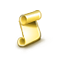684

2017.06.05 13:03

```//+----------------------------------------------+
//| 脚本程序的输入参数                 |
//+----------------------------------------------+
input double  MM=0.1;       // 资金管理
input int  DEVIATION=10;    // 价格偏移
input uint  LEVEL=300;      // 设置订单的距离点数
input uint  STOPLEVEL=150;  // 止损限制的距离点数
input uint  STOPLOSS=100;   // 止损价距离当前价格的点数
input uint  TAKEPROFIT=800; // 获利距离当前价格的点数
input uint RTOTAL=4;        // 如果交易不成功的尝试次数
input uint SLEEPTIME=1;     // 在尝试之间暂停的秒数
```OpenBuyStopLimitOrderExp_Stochastic_Chaikins_Volatility

Exp_Stochastic_Chaikins_Volatility EA交易是基于 Stochastic_Chaikins_Volatility 指标颜色变化的。OpenBuySellStopLimitOrdersEXP_MAX_LOT